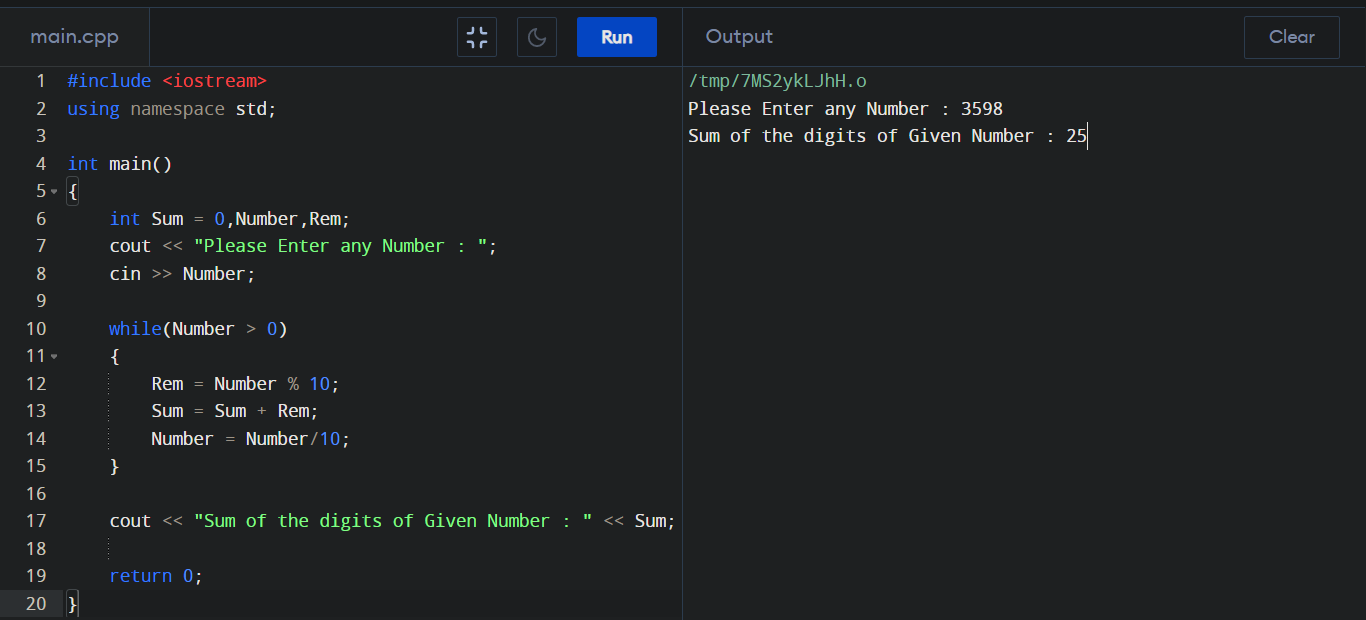C++ Program to Calculate Sum of Digits

To get sum of each digits in C++ program here we used relational operator for entering in loop and 'cout' for showing result .

Sum of Digits in C++ :

#include <iostream> using namespace std; int main() { int Sum = 0,Number,Rem; cout << "Please Enter any Number : "; cin >> Number; while(Number > 0) { Rem = Number % 10; Sum = Sum + Rem; Number = Number/10; } cout << "Sum of the digits of Given Number : " << Sum; return 0; }

Output :